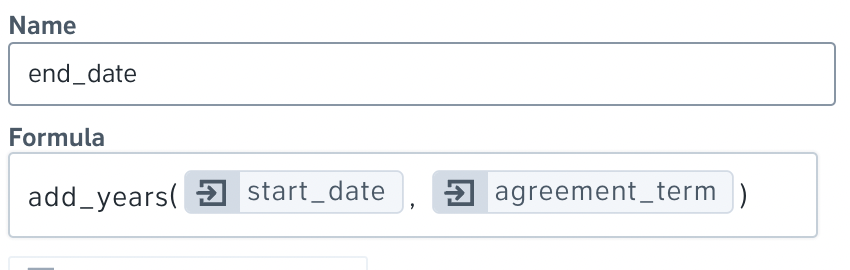Help Center
1. Help Center
2. FAQs & Troubleshooting
3. Documents & automated templates

# How do I use formulas in automated templates?

## Learn how to set up automated calculations in templates using formulas

A formula is a mathematical relation between values giving a certain result. In Precisely, you're able to create formulas for any value that can be calculated based on previously given answers.

• Example 1: You're able to create a formula to automatically count the end date of your agreement based on the start date and the duration of the agreement.
• Example 2: You're able to automate the yearly salary based on a monthly amount, and if applicable, include a salary bonus in the final calculation.

### Why use formulas?

Formulas enable users to automate their contracts even more and work smarter and more efficiently. By using formulas in Precisely templates, you will

1. Draft contracts faster by eliminating unnecessary questions in the drafting process,
2. Avoid the actual need to calculate a value on your own, and
3. Minimize the risk of making a mistake in calculations (happens to the best of us🥲)

Once the project is generated, the result of the formula will be added to the document in the same way as the questionnaire answers. The only and the main difference is that formulas will always be automatically calculated.

### How to create a formula?

To create a formula, simply

1. Select the wanted template and open the document in the Template editor.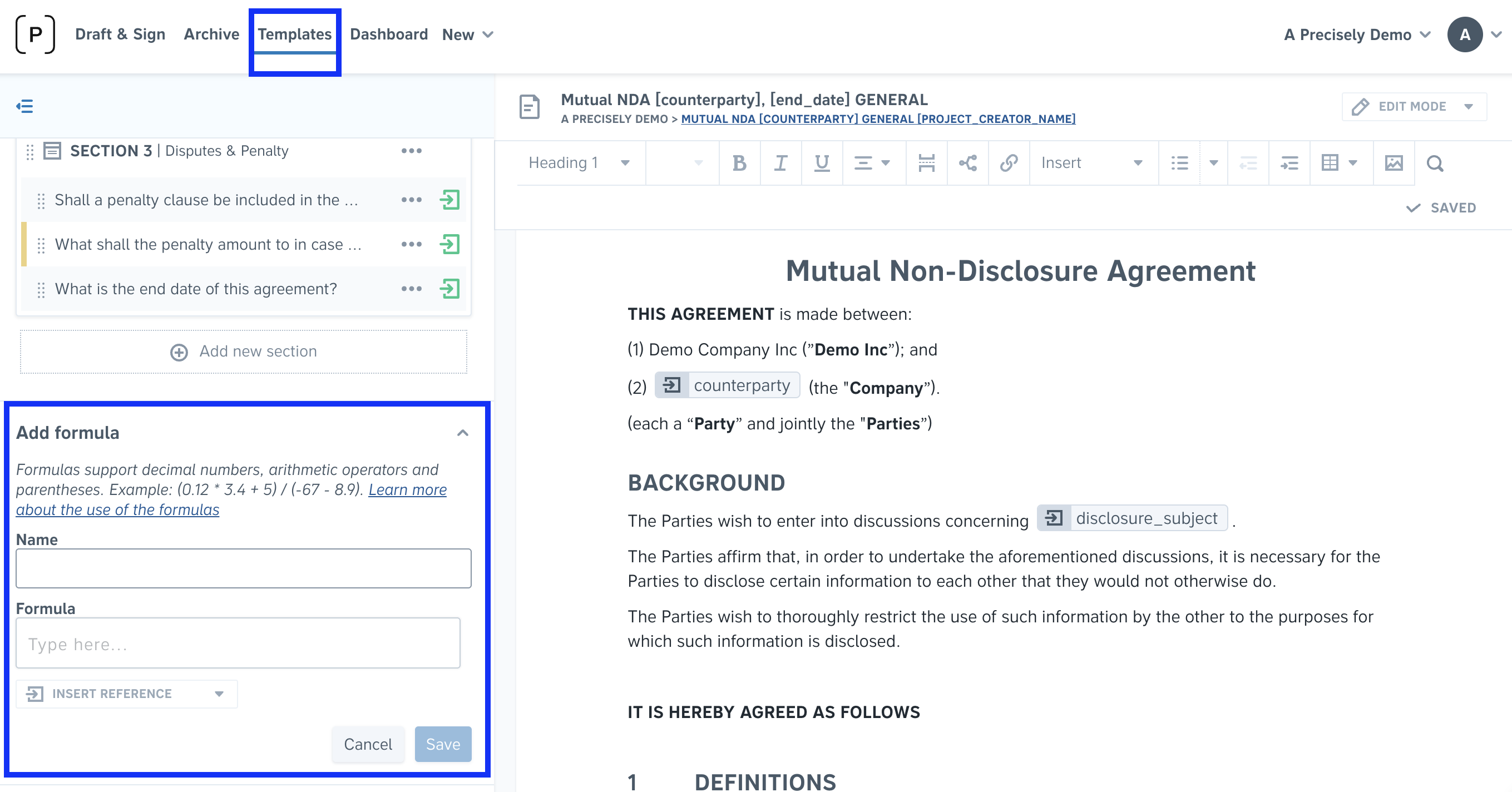2. Set a reference name for the formula and create the calculation by inserting numbers, references, and operators. Some of the allowed operators are:
( )   Parentheses
-     Subtraction
*     Multiplication
/     Division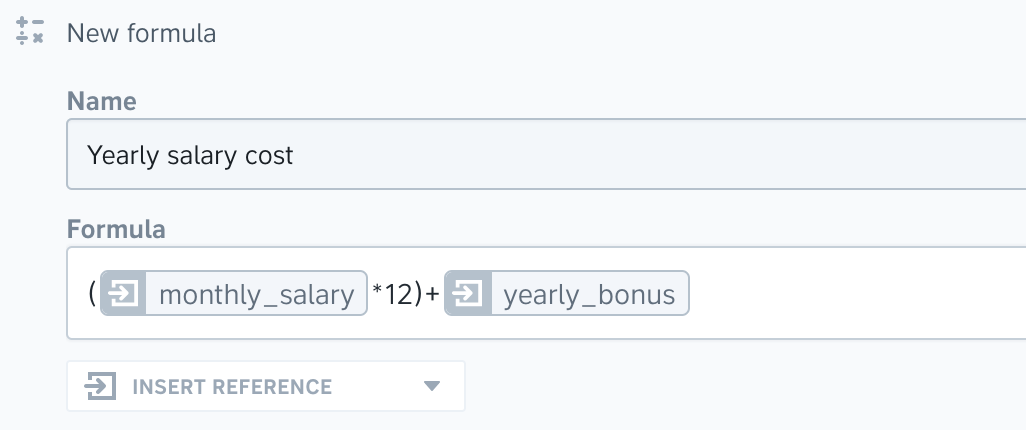Keep in mind! In order for the formula to work and calculate as expected:

• a reference containing numbers must have a numeric type of answer. Make sure to write decimal numbers using decimal dots. However, in the generated document, the number will appear with a decimal comma.
• 4.5 - 4,5
• a reference containing a date must have a date type of answer.
• if a reference has a multiple choice or a short text type of answer, the answer is always treated as a text, even if there's an actual number. In this case, you must use double quotation marks.
• "Yes" - Yes

### Examples of formulas

Not being aware of the formulas and their powerful impact often results in creating and answering unnecessary extra questions, calculating on your own, or setting up several conditional blocks. All of that instead of simply creating a formula. And don't worry, you only need to set this up once - and then use it forever. Besides that, the Precisely Team can always help!

Here are some examples of frequently used formulas:

#### Calculating value, cost or salary

These formulas are often used in e.g. employment agreements or different purchase contracts.

Example:

Question (NUMERIC): What is the monthly salary of the employee?

Formula below output: 30000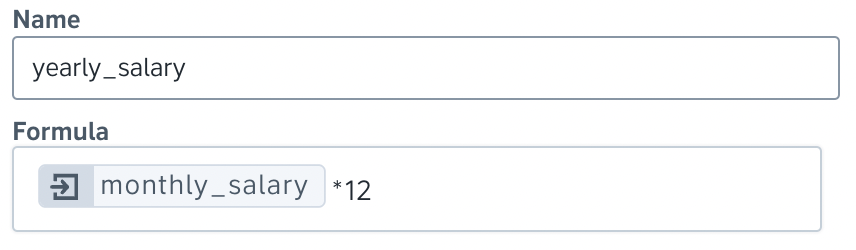Formula: reference*12

Example:

Question 1 (NUMERIC): What is the price per unit?

Question 2 (NUMERIC): How many units are included?

Formula below output: 3000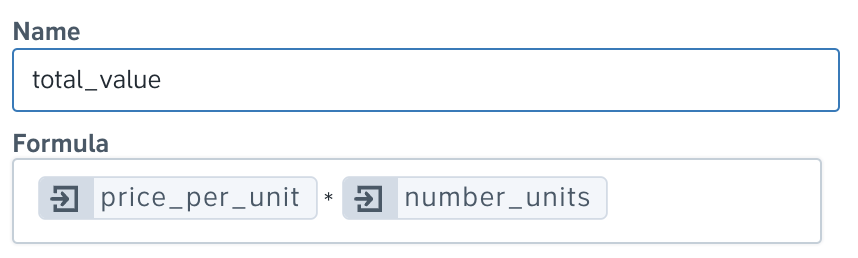Formula: reference1*reference2

#### Calculating date

Date formulas are mostly used to calculate a date based on the already given date and the duration of the contract. There are different ways of doing this depending on if there is a set amount of time between the dates or if that depends on the answer to another question.

The quickest way is to already know there is a specific amount of time until the contract is expired - for example, one year or three months. In this way, you can already set this up in the formula, without needing to create any additional questions.

Example (one-year contract validity):

Question (DATE): What is the start date of this agreement?

Formula below output: 2023-01-01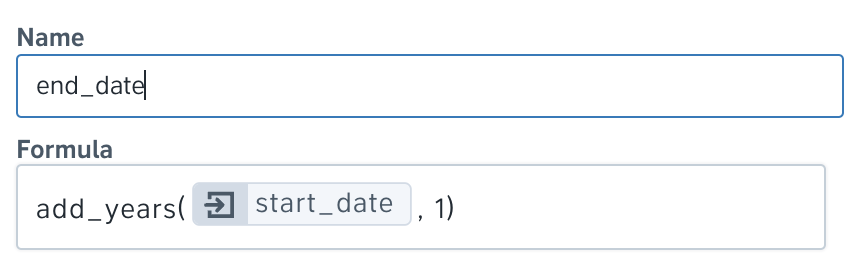Example (three months contract validity):

Question (DATE): What is the start date of this agreement?

Formula below output: 2022-04-01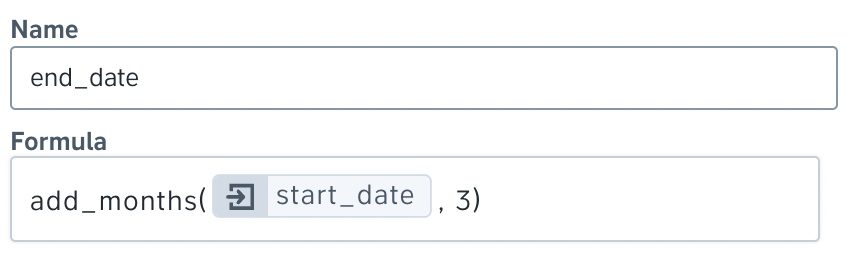On the other hand, if the contract duration is variable depending on a case, an additional question about the length should be created.

Example:

Question 1 (DATE): What is the start date of this agreement?